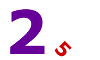# Elementary Math Lessons

Elementary math includes number theory, which is the study of whole numbers and relations between them. This unit includes in-depth instruction on factors, multiples, primes, composites, divisibility tests, and exponents. Try our elementary math lessons below, or browse other instructional units.Elementary Math Description Factors and GCF To define factor and greatest common factor. To find all factors of a whole number. To find the GCF of two or more whole numbers. Multiples and LCM To define multiple and least common multiple. To find multiples of whole numbers. To find the LCM of two or more whole numbers. Prime and Composite Numbers To define primes and composites. To determine if a whole number is prime or composite by examining its factors. Divisibility Tests To determine if larger whole numbers are prime or composite by using divisibility tests for 2, 3, 4, 5, 6, 9 and 10. Exponents To define exponent, base and power. To learn exponential notation. To read and write numbers in exponential form. To convert between standard, factor and exponential form. Patterns and Exponents To recognize patterns with exponents. To predict the next term in a sequence of numbers written in exponential form. Practice Exercises To complete 10 additional exercises as practice with number theory. To assess students' understanding of all theory presented. Challenge Exercises To solve 10 additional problems that challenge students' understanding of all theory presented. To hone students' problem-solving skills. Solutions To review complete solutions to all exercises in this unit number theory unit. Learning Objectives Learn about factors, multiples, GCF, LCM, prime and composite numbers, divisibility, exponents, and patterns with exponents.